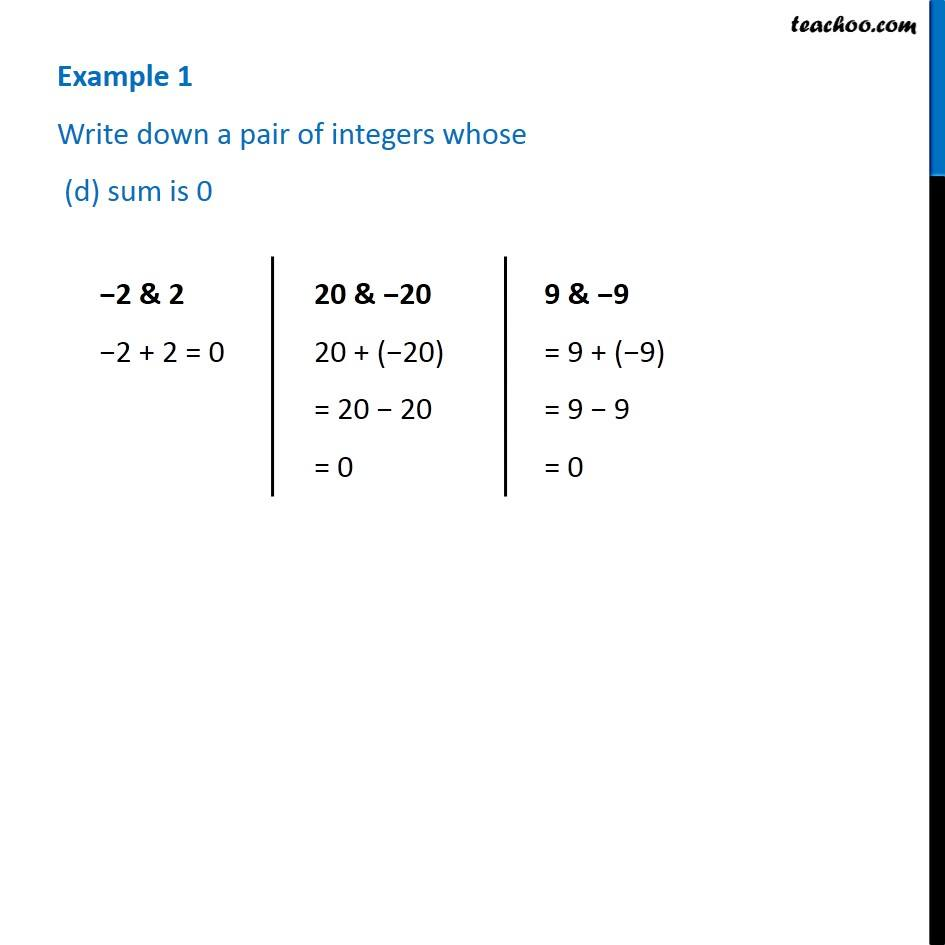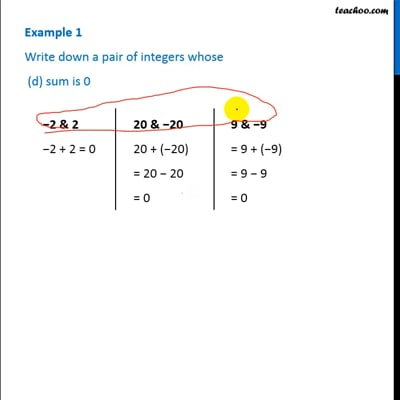Examples

Chapter 1 Class 7 Integers
Serial order wiseThis video is only available for Teachoo black users

Introducing your new favourite teacher - Teachoo Black, at only ₹83 per month

### Transcript

Example 1 Write down a pair of integers whose (d) sum is 0−2 & 2 −2 + 2 = 0 20 & −20 20 + (−20) = 20 − 20 = 0 9 & −9 = 9 + (−9) = 9 − 9 = 0